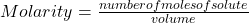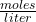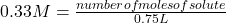What is the amount of moles of NaOH, if the solution molarity is .33 M NaOH in 0.75 L solution

Question

What is the amount of moles of NaOH, if the solution molarity is .33 M NaOH in 0.75 L solution

in progress 0
5 months 2021-08-28T08:01:57+00:00 2 Answers 6 views 0

The amount of moles of NaOH is 0.0495

Explanation:

Molarity is a measure of concentration that is defined as the number of moles of solute that are dissolved in a given volume.

In symbolic form molarity  is presented as:Molarity is expressed in units.

In this case:

• Molarity= 0.33 M
• Number of moles of solute= ?
• Volume= 0.75 L

Replacing in the definition of molarity:number of moles of solute= 0.33 M* 0.75 L

number of moles of solute= 0.0495 moles

The amount of moles of NaOH is 0.0495

0.248 moles of NaOH

Explanation:

Concept of Molarity is mol/L

[x] = 1 M

1 mol of x contained in 1 L of solution. We write molar concentrations between []

M = mol/L then M . L = mol

Our solution is NaOH

0.33 M . 0.75L = mol

This solution contains 0.248 moles of NaOH